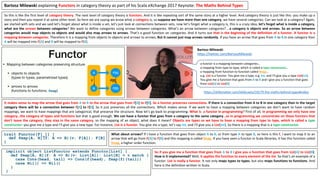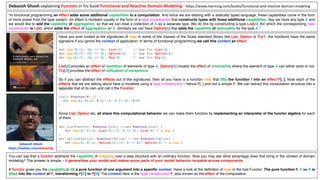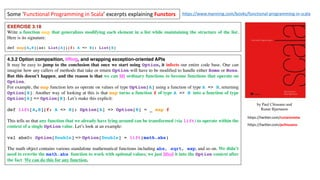Successfully reported this slideshow.×
1 of 5

Functors

2

Share

What would be a good answer to the question of what functors are? To help formulating a reasonably well rounded answer, I have made some slides capturing what I considere particularly useful aspects of the following sources’ exposition of the concept of Functor:
* Bartosz Milewski’s Scala eXchange 2017 Keynote, The Maths Behind Types
*Paul Chiusano and Runar Bjarnason’s book, Functional Programming in Scala
* Debasish Ghosh’s book - Functional and Reactive Domain Modeling
* Christopher Allen, and Julie Moronuki's book - Haskell Programming from First Principles

See all

See all

Functors

1. 1. So this is like the first level of category theory. The next level of category theory is functors. And it is like repeating sort of the same story at a higher level. And category theory is just like this: you make up a story and then you repeat it at some other level. So here we are saying we know what a category is, so suppose we have more than one category, we have several categories. Can we look at a category? Again, we started with sets and we said let’s forget about what is inside a set, let’s just look at connections between sets, now let’s forget what a category is, this is a crazy idea, let’s forget what is inside a category, what are the arrows between categories? We want to define categories using arrows between categories. What’s an arrow between categories? …a category is objects and arrows. So an arrow between categories would map objects to objects and would also map arrows to arrows. That’s a good function on categories. And it turns out that is the beginning of the definition of a functor. A functor is a mapping between categories. Therefore it is a mapping from objects to objects and arrows to arrows. But it cannot just map arrows randomly. If you have an arrow that goes from A to B in one category then A will be mapped into F[A] and B will be mapped to F[B]. It makes sense to map the arrow that goes from A to B to the arrow that goes from F[A] to F[B]. So a functor preserves connections. If there is a connection from A to B in one category then in the target category there will be a connection between F[A] to F[B]. So it just preserves all the connections. Which makes sense. If we want to have a mapping between categories we don’t want to have random mappings, we want to have mappings that are categorical, that preserve the structure. Now let’s go back to programming. What is a functor in programming? First of all, in programming we only have one category…the category of types and functions but that is good enough. We can have a functor that goes from a category to the same category…so in programming we concentrate on these functors that don’t leave the category, they stay in the same category, so the mapping of an object, what does it mean? Objects are types so we have to have a mapping from type to type, which is called a type constructor: you give me a type and I’ll give you a new type. For instance, List is a functor. You give me a type, let’s say Int, and I’ll give you a List[Int]. So there is a mapping that is a type constructor. What about arrows? If I have a function that goes from object A to B, or from type A to type B, so here is this f, I want to map it to an arrow that will go from F[A] to F[B] and this mapping is called fmap. If you have seen a functor in Scala libraries, it has this function called fmap, a higher order function. So if you give me a function that goes from A to B I give you a function that goes from List[A] to List[B]. How is it implemented? Well, it applies the function to every element of the list. So that’s an example of a functor. List is really a functor. It not only maps types to types, but also maps functions to functions. And here is the definition written in Scala. Bartosz Milewski https://twitter.com/BartoszMilewski Bartosz Milewski explaining Functors in category theory as part of his Scala eXchange 2017 Keynote: The Maths Behind Types a functor is a mapping between categories… a mapping from type to type, which is called a type constructor… a mapping from function to function called fmap… e.g. List is a functor. You give me a type, e.g. Int, and I’ll give you a type List[Int]. You give me a function that goes from A to B and I give you a function that goes from List[A] to List[B] https://skillsmatter.com/skillscasts/10179-the-maths-behind-types#video